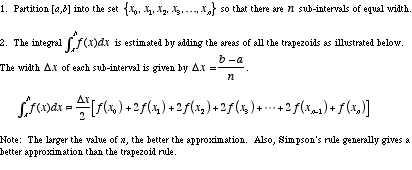# trapezoid rule

Trapezoid Rule

A method for approximating a definite integralusing linear approximations of f. The trapezoids are drawn as shown below. The bases are verticallines.

To use the trapezoid rule follow these two steps: